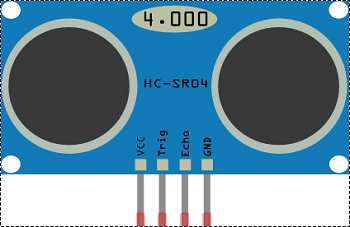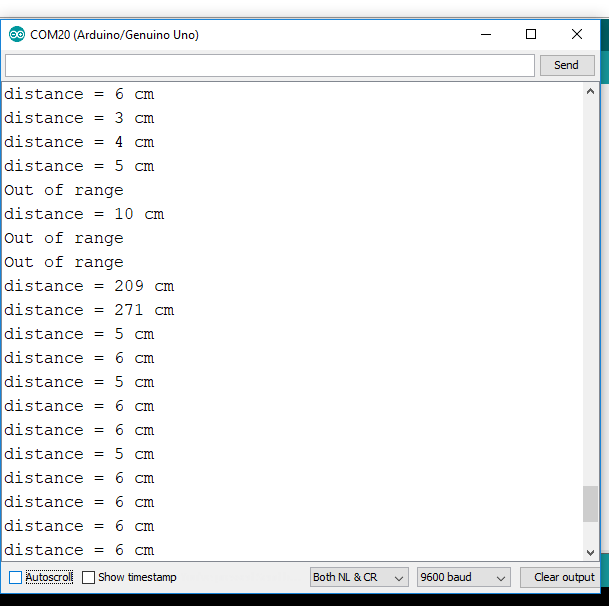# Ultrasonic sensor with Arduino

In this tutorial, we will see how to interface ultrasonic sensor with arduino and how to get its output on the serial monitor.

The ultrasonic sensor is a sensor which emits ultrasonic sound waves from its transmitter and this sound wave returns back to the receiver of the sensor after reflecting from an obstacle or surface and calculates the total time taken by the wave to get return.

We know the velocity of sound is 343 m/s & if we can get time taken by the wave to travel, then, we can easily calculate distance between the sensor and the obstacle.

## ULTRASONIC SENSOR HAS 4 PINS

1. VCC
2. Trig – Trigger
3. Echo
4. GND – GroundIn simple terms, we can say Trig is a transmitter and the Echo is a receiver.

Generally Ultrasonic sensor is used in applications where measuring distance and/or sensing objects is required. The sensor works with the simple formula that is

#### Distance = Speed × Time

The Ultrasonic transmitter transmits ultrasonic wave pulse, this wave travels in the air and when it gets objected by any material or obstacle it gets reflected back toward the sensor or in other words when an obstacle detected waves reflects back towards the sensor, this reflected wave is observed by the Ultrasonic receiver see picture below

Now, to calculate the distance using the above formula, we should know the Speed and time. Since we are using the Ultrasonic wave we know the universal speed of US wave at room conditions which is 343 m/s.

The circuitry inbuilt on the module will calculate the time taken for the ultrasonic wave to come back where the echo pin turned high for that same amount of time, this way we can also know the time taken.

### ULTRASONIC SENSOR PIN CONFIGURATION

 Pin Number Pin Name Description 1 Vcc The Vcc pin powers the sensor, typically with +5V 2 Trigger Trigger pin is an input pin. This pin has to be kept high for 10 us to initialize measurement by sending US waves. 3 Echo The echo pin is an output pin. This pin goes high for a period of time which will be equal to the time taken for the US wave to return back to the sensor. 4 Ground This pin is connected to the Ground of the system.

### HC-SR04 SENSOR FEATURES

• Operating voltage: +5 V
• Theoretical  Measuring Distance: 2 cm to 400 cm
• Practical Measuring Distance: 2 cm to 80 cm
• Accuracy: 3 mm
• Measuring angle covered: <15°
• Operating Current: <15 mA
• Operating Frequency: 40 Hz

## DETAILED WORKING OF ULTRASONIC SENSOR

• The current consumed by the sensor is less than 15 mA and hence can be directly powered by the on-board 5 V pins (If available).
The Trigger and the Echo pins both are I/O pins and hence they can be connected to I/O pins of the microcontroller. Connect the ground pin of the sensor to the ground pin of the arduino similarly VCC pin to 5V.
• Its working is quite simple, as discussed above, it has a trigger and an echo pin.• A signal of +5 V (HIGH) is sent over the Trigger pin for around 10 microseconds in order to trigger the sensor. When an ultrasonic sensor gets a trigger signal on its trigger pin then it emits an ultrasonic wave at a frequency of  40 KHz from the transmitter. As a signal transmitted, after 10 microseconds we simply provide a LOW signal to the trigger pin of the sensor to turn it off.
• After turning OFF triggering the sensor we will give HIGH to the echo pin of the sensor it will remain HIGH till it receives the reflected signal/wave back, as receiver receives signal then echo pin turns automatically OFF.
If it does not get a signal back in a fixed interval of time then it assumes there is no obstacle and turns automatically OFF and waits for the next cycle of triggering.
The amount of time during which the Echo pin stays high is measured by the Arduino/ MCU/ MPU as it gives the information about the time taken for the wave to return back to the Sensor. Using this information the distance is measured.
• And this process continued.

This action will trigger an ultrasonic wave at a frequency of 40 Hz from the transmitter and the receiver will wait for the wave to return.

## HARDWARE REQUIRED TO INTERFACE ULTRASONIC SENSOR WITH ARDUINO

Following Hardware will be required to perform this circuit.

 S.No. Item Quantity 1. Arduino Uno 1 2. Breadboard 1 3. Ultrasonic Sensor 1 4. LED 1 5. Resistor 220 ohm 1 6. Male to Male jumper 6

### BUILDING CIRCUIT

 Arduino Ultrasonic HC SR04 8 Trig 7 Echo 5V 5V GND GND

## ULTRASONIC SENSOR ARDUINO CODE

In this, we are using Serial monitor not LCD so if you are new to arduino and not aware of Serial refer this link serial communication in arduino.

```// defining pins and variables
long duration;
int distance, led = 3;
const int trigPin = 8, echoPin = 7;

// setup pin mode and begins serial communication with baud rate 9600
void setup() {
pinMode(led, OUTPUT);
digitalWrite(led, LOW);
delay(100);
pinMode(trigPin, OUTPUT);
pinMode(echoPin, INPUT);
Serial.begin(9600);
}

void loop() {
// calling a user defined function "calculateDistance()" which returns distance in integer form
distance = calculateDistance();

// if distance is less than 20 light up led and print distance as well
if (distance < 20) {
digitalWrite(led, HIGH);
Serial.print("distance = ");
Serial.println(distance);
delay(500);
}

// if distance is greater than 20 and less than 400 turn Off led and print distance as well
else if (distance > 20 && distance <= 400) {
digitalWrite(led, LOW);
Serial.print("distance = ");
Serial.println(distance);
delay(500);
}

// if distance is less than 2 and greater than 400 print message "Out of Range"
else if (distance < 2 || distance > 400) {
Serial.println("Out of range");
}
}

/* defining a function calculateDistance() of int type, which means it must return the value of int type.
then set trigger Pin LOW for 2 microseconds, so that no noise present initially
then set trigger Pin HIGH for 10 us, so that ultrasonic sensor transmit a sound wave of 40KHz for 10 us as in datasheet
then set trigger Pin LOW and immediately without delay call a function pulseIn and make echo Pin high which returns time and stored in variable duration
then convert it by speed formula and returns the distance where function calculateDistance() called
*/
int calculateDistance() {
digitalWrite(trigPin, LOW);
delayMicroseconds(2);
digitalWrite(trigPin, HIGH);
delayMicroseconds(10);
digitalWrite(trigPin, LOW);
duration = pulseIn(echoPin, HIGH);
distance = duration * 0.034 / 2;
return distance;
}
```

#### OUTPUT

When an obstacle is present in the range of 2 – 400 cm then the sensor can detect it and if it reaches to 40 cm apart from the sensor we can turn ON led accordingly to get aware of it.## APPLICATIONS

• Used to avoid and detect obstacles with robots like biped robot, obstacle avoiding robot, path finding robot etc.
• Used to measure the distance within a wide range of 2 cm to 400 cm
• Can be used to map the objects surrounding the sensor by rotating it
• Depth of certain places like wells, pits etc. can be measure, since the waves can penetrate through the water

5 1 vote
Article Rating
Subscribe
Notify of